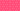PricingResources

# What Is Notional Value?

Written by

If you’re involved with foreign currency trades, futures contracts, and interest rate swaps, you’ve probably seen references to a position’s notional value. So, what is notional value, and how is it used in derivatives trading? Here’s a quick rundown of how it works.

## What is notional value and financial leverage?

Used in derivatives trading, notional value is a term referring to the value of an underlying asset. It might be used in relation to contracts in the futures, currency, or options markets, describing the position’s total value or contract amount. Also called the notional principal amount or notional amount, notional value is simply the face value of a security used to calculate payments. It shows you how much money is controlled by the investment position.

With financial leverage, you can use a smaller amount of money to control a larger position, defined by its notional value. To use financial leverage ratios, you can simply divide notional value by market value. Here’s a financial leverage ratio formula to illustrate this concept:

A derivative contract’s notional value will be higher than its market value because of leverage. While the market value is the price that you can buy or sell the position in the marketplace, notional value is the total underlying value of a contract or position. Notional value is more theoretical than market value, which pertains more to the security’s actual price.

## How to calculate notional value

Each derivatives contract comes with unique multipliers, weights, volumes, or other factors to describe the underlying asset. One example would be a single gold futures contract unit with a weight of 100 troy ounces. The notional value would be 100 times the market price of gold, according to the notional value formula:

When an investor wishes to purchase a derivatives contract, they’ll pay at this predetermined price. For example, if someone purchased a futures contract for gold at 10 units, this would be multiplied by the 100 for a total notional value of \$1000. However, due to the concept of leverage, the investor wouldn’t need to pay the full notional value. Instead, they would pay the market value or initial margin instead.

In other words, when purchasing forward contracts, you must multiply units by price to determine the notional value or spot price.

## How is notional value used?

Notional value is an important reference point for investors, particularly in assessing risk. Investors and analysts might use notional value to calculate hedge ratios and offset portfolio risk. Written as a formula, here’s how you would use notional value and risk in calculating hedge ratios:

The notional value meaning is useful not only for futures contracts as described above, but it can also be used for things like equity options, foreign currency derivatives, interest rate swaps, and any other contract involving an underlying asset price.

For example, in the case of interest rate swaps the notional value meaning would refer to the pre-specified value that the interest rate payment exchange takes place. This figure is important because it will be the basis for any interest payments. Each counterparty agrees upon a contract to exchange interest payments, calculated on notional principal value.

In the case of foreign currency derivatives, there would be two notional values to consider – one for each currency that you’re exchanging.

## The bottom line

When trading derivatives, it’s important to look both at notional value and market value to work out the level of risk vs reward. You can then use this calculation to compare different investment options by their underlying values.

#### We can help

GoCardless helps you automate payment collection, cutting down on the amount of admin your team needs to deal with when chasing invoices. Find out how GoCardless can help you with ad hoc payments or recurring payments.

Sales

Support

+61 3 8375 9198